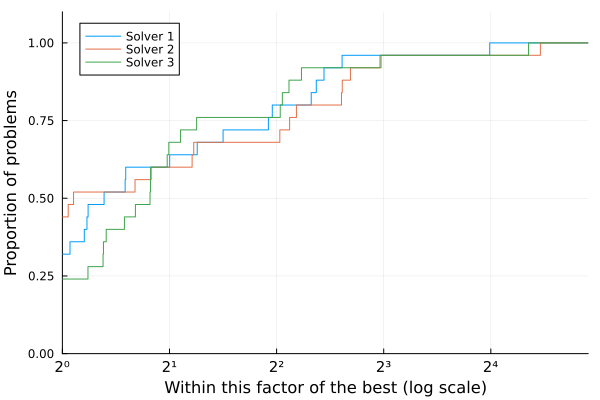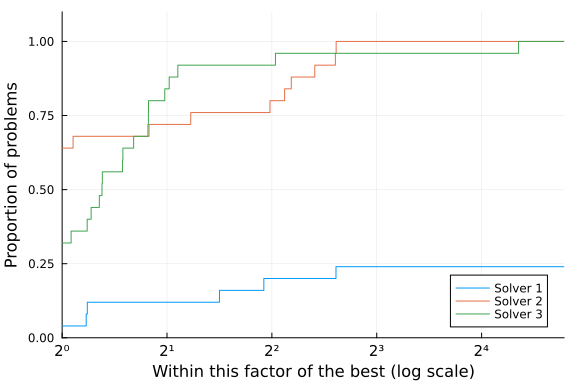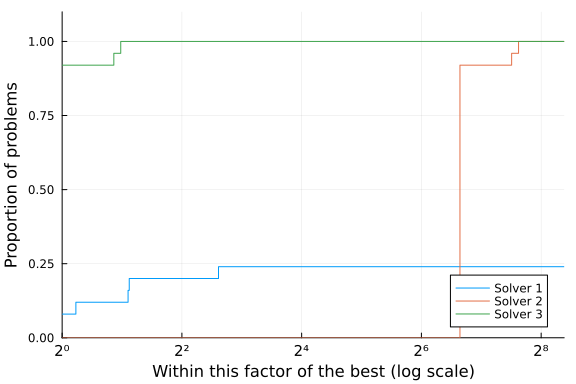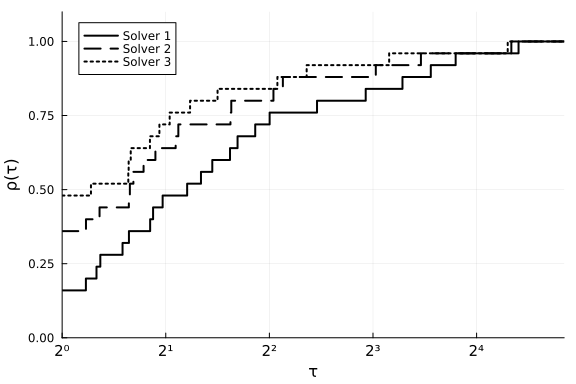# Introduction to BenchmarkProfilesThis tutorial is essentially a collection of examples.

## Performance Profile

Performance profiles are straightforward to use. The input is a matrix `T` with entries `T[i,j]` indicating the cost to solve problem `i` using solver `j`. Cost can be, for instance, elapsed time, or number of evaluations. The cost should be positive. If any cost is zero, all measures will be shifted by 1.

Basic usage:

``````using BenchmarkProfiles, Random, Plots

Random.seed!(0)

T = 10 * rand(25, 3)
performance_profile(PlotsBackend(), T, ["Solver 1", "Solver 2", "Solver 3"])``````A negative or infinite cost indicates a failure:

``````T[2:20,1] .= Inf
performance_profile(PlotsBackend(), T, ["Solver 1", "Solver 2", "Solver 3"])``````Here's an example with a strongly superior solver.

``````T[:,2] = 100T[:,3]
performance_profile(PlotsBackend(), T, ["Solver 1", "Solver 2", "Solver 3"])```````Plots` arguments can be passed to `performance_profile()` or used as they normally would be with `Plots`. In the example below, we pass `xlabel` to `performance_profile` and set `ylabel` through `ylabel!`.

``````using Plots

T = 10 * rand(25, 3)
performance_profile(PlotsBackend(), T, ["Solver 1", "Solver 2", "Solver 3"],
lw=2, c=:black, linestyles=[:solid, :dash, :dot], xlabel="τ")
ylabel!("ρ(τ)")``````